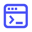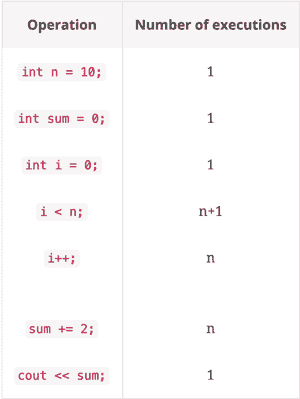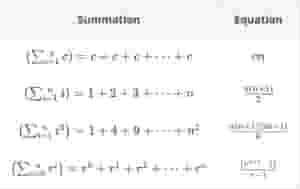Home/Blog/The insider's guide to algorithm interview questions

# The insider's guide to algorithm interview questions

Feb 18, 2022 - 7 min readEducativeNote: This post was originally published in 2019 and has been updated as of Feb. 18, 2022.

Algorithms are a big part of coding interviews. Whether your programming language is Python or Java, or you’re interviewing at Microsoft or Amazon, you’ll surely be asked programming interview questions to test your knowledge of data structures, algorithms, and optimization.

Today, we’ll take a look at some common algorithms that you’ll need to know for a software development interview. We’ll also cover some practice problems.

Here’s what we’ll cover today:

#### Get hands-on with algorithms today.

Try one of our 300+ courses and learning paths: Grokking the Coding Interview.

## 3 steps to prepare for your interview

There’s a lot of advice out there for how to prepare that can become overwhelming. To simplify it, try focusing on meeting these 3 steps:

1. Remember to revise the basics.
2. Know the implementation and the pros/cons for each of the algorithmic paradigms.
3. Understand how to measure and optimize your program with asymptotic analysis (Big O).

These are essential, timeless skills that will help you in every interview. To strengthen them, you’ll want to practice using different algorithms with coding questions.

## What are algorithmic paradigms?

In computer science, algorithmic paradigms are general approaches to the construction of efficient solutions to problems. In other words they are a method, strategy, or technique to solving a problem and are essential for every programmer, no matter their programming language.

These are often tested in interviews so it’s worth spending extra time reviewing each.

Algorithmic paradigms are great because they lay down a framework suited for solving a broad range of diverse problems.

For example: Backtracking

This paradigm involves solving problems by trying to build a solution incrementally, one piece at a time, removing those solutions that fail to satisfy the constraints of the problem.

### What algorithms should I be aware of for my interview?

Algorithm questions can cover a broad range of topics.

• Brute Force — This method requires us to go through all the possibilities to find a solution to the problem we are meaning to solve. This is often the algorithm that comes to mind first, and even though it may be the least efficient, you’re guaranteed a solution.
• Greedy algorithms — an algorithmic paradigm that builds up a solution piece by piece, meaning it chooses the next piece that offers the most obvious and immediate benefit.
• Dynamic programming — an optimization algorithm for recursive functions. Using dynamic programming, you can store the results of subproblems, so that we do not have to re-compute them when needed later.
• Divide and conquer — a pattern that breaks the problem into smaller subproblems that are then solved recursively and finally reassembled. Often used for sorting operations.
• Sorting and searching algorithmsmergesort, quicksort, selection sort, insertion sort, and bubble sort algorithms
• Graph algorithms — breadth first graph traversal, depth first graph traversal

## Measuring efficiency

Each of these algorithms has a different efficiency. You’ll often be asked to find the efficiency or “complexity” an algorithm you wrote or to improve one given to you. To do this, you’ll need to use asymptotic analysis.

Complexity is an approximate measure of the efficiency of an algorithm and is associated with every algorithm you write. This is something that all programmers must be constantly aware of.

There are two kinds of complexities: time and space. Time complexity and space complexity are essentially approximations of how much time and how much space an algorithm will take to process certain inputs respectively.

Typically, there are three tiers to solve for:

• Best case — represented as Big Omega or Ω$(n)$
• Average case — represented as Big Theta or Θ$(n)$
• Worst case — represented as Big O Notation or $O(n)$

Big O is preferred to analyze an algorithm, as average and best cases do not give insight to the efficiency of an algorithm for most use-cases.

## Big O complexity

If you’re in an interview and are asked to find the Big O complexity of an algorithm here is a general rule of thumb:

• Drop the leading constants
• Ignore the lower order terms

Example: Find the Big O complexity of an algorithm with the time complexity $3n^3 + 4n + 2$

This simplifies to $O(n^3)$.

### How to calculate complexity without a given equation

There are three steps you’ll want to take when calculating the time complexity of an algorithm:

• List down all the basic operations in the code
• Count the number of times each gets executed
• Sum all the counts to get an equation

Here’s a simple example that measures the time complexity of a for loop of size n.

Here is a loop of size n:

#include <iostream>using namespace std;int main(){  int n = 10;  //  0(1)  int sum = 0; // 0(1)  for (int i=0; i<n; i++)    sum+=2; // 0(1)  cout << sum; // 0(1)  return 0;}

First, split the code into individual operations and then compute how many times it is being executed, which will look like this:After counting how many times each operation is executing, you just add all of these counts to get the time complexity of this program.

Time Complexity =

$1 + 1 + 1 + (n + 1) + n + n + 1 = 3 + (n + 1) + 2n + 1 => 3n + 5$

General tips for asymptotic analysis:

• Every time a list or array gets iterated over x length times, it is most likely in $O(n)$ time.
• When you see a problem where the number of elements in the problem space gets halved each time, it will most probably be in $O(logn)$ runtime.
• Whenever you have a singly nested loop, the problem is most likely in quadratic time.

Useful formulae for calculating time complexity of an algorithm:#### Get hands-on with algorithms today.

Try one of our 300+ courses and learning paths: Grokking the Coding Interview.

## Sample problems

• Asymptotic analysis: Compute the Big O complexity of the code snippet given below.
int main(){   int n = 10;   int sum = 0;   float pie = 3.14;      for (int i=1; i<n; i+=3){     cout << pie << endl;     for (int j=1; j<n; J+=2){       sum += 1;       cout << sum << endl;     }   }}
• Sorting and searching algorithms: Implement a function that takes two sorted arrays of variable length and finds the median of the two arrays.

• Graph algorithms: Implement a function that returns the number of nodes at a given level of an undirected graph.

• Greedy algorithms: Given an infinite number of quarters (25 cents), dimes (10 cents), nickels (5 cents), and pennies (1 cent), implement a function to calculate the number of COINS to represent V cents.

• Dynamic programming algorithms: A child is running up a staircase with n steps and can hop either 1 step, 2 steps, or 3 steps at a time. Implement a function to count the number of possible ways that the child can run up the stairs.

• Divide and conquer algorithms: Given a 2D array of k rows and 4 sorted columns and an empty 1D output array of size k∗n, copy all the elements from k sorted arrays to the k∗n output array using a divide and conquer approach.

## Next steps

As a software engineer, you can ace rock your programming interviews by showcasing your knowledge of algorithms. As always, hands-on practice of programming questions is the best way to prepare for your interviews.

To help you ace your programming interview, we’ve created the Grokking the Coding Interview course. This course covers both algorithm interview questions and data structure interview questions. You’ll practice real-world challenges and tutorials to test your understanding. You’ll learn sort algorithms as well as breadth-first search (BFS) and depth-first search (DFS) algorithm. You’ll also get hands-on practice doing various operations on binary search trees, linked lists, and hash tables.